What does it do?

Calculates the compound interest

Formula breakdown:

=FV(rate, nper, pmt, [pv])

What it means:

=FV(interest rate, number of periods, periodic payment, initial amount)

Say that you turn 18 years today (CONGRATS!) and you find out that your parents deposited an amount with their bank when you were born.

Now that you are 18 years old you can collect this money and go spend it all in one day!

How much would be available for you to spend?

Thankfully there is an easy way to calculate this with Excel’s FV formula! FV stands for Future Value.

The future value (FV) is the value of a current asset at a specified date in the future based on an assumed rate of growth over time.In our example below, we have the table of values that we need to get the compound interest or Future Value from:

(Change the NUMBER OF YEARS column to 18 to see the results on your 18th birthday)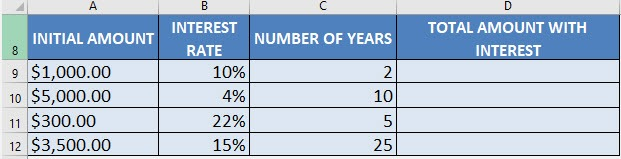I explain how you can do this below:

STEP 1: We need to enter the FV function in a blank cell:

## =FV(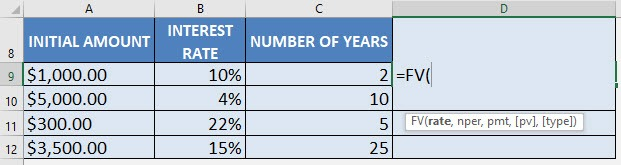STEP 2: The FV arguments:

## rate

What is the rate of the interest?

Select the cell containing the interest rate (make sure that this is in a percentage):

## =FV(B9,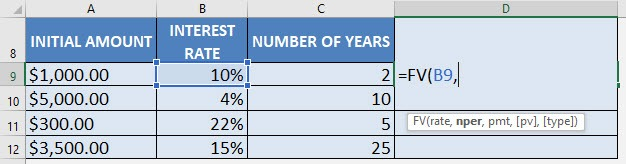## nper

How many periods?

Select the cell containing the number of years:

## =FV(B9, C9,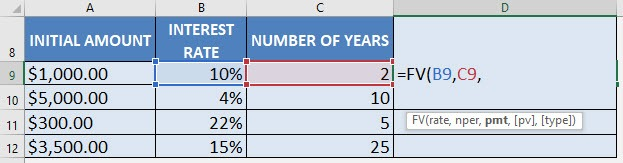## pmt

What is the periodic payment?

We have no periodic payment, only an initial amount, so let us put in 0:

## =FV(B9, C9, 0,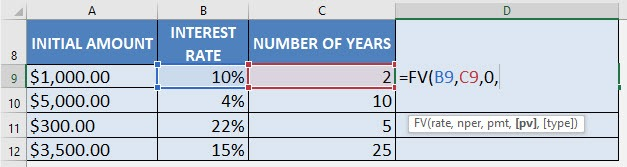## pv

What is the initial amount?

PV stands for present value, the initial amount. We need to change this to a negative value by multiplying -1.

The reason why we need this as a negative value as Excel treats this as “money out” for your investment.

## =FV(B9, C9, 0, A9 * -1)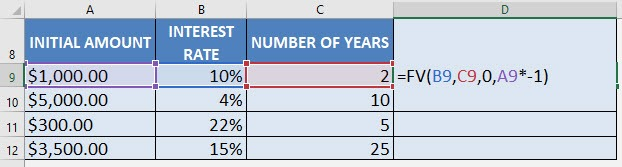Apply the same formula to the rest of the cells by dragging the lower right corner downwards.

You now have all of the compound interest results!  GO OUT & SPEND!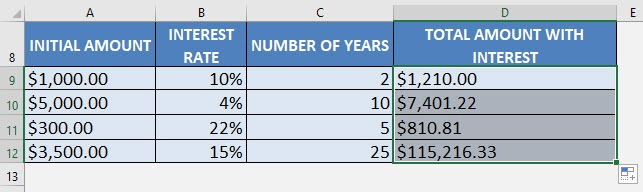Get the Compound Interest with Excel’s FV Formula